### JPL's Wireless Communication Reference Website

#### Chapter: Wireless Channels Section: Multipath fadingRayleigh fading is caused by multipath reception. The mobile antenna receives a large number, say N, reflected and scattered waves. Because of wave cancellation effects, the instantaneous received power seen by a moving antenna becomes a random variable, dependent on the location of the antenna.A sample of a Rayleigh fading signal. Signal amplitude (in dB) versus time for an antenna moving at constant velocity. Notice the deep fades that occur occasionally. Although fading is a random process, deep fades have a tendency to occur approximately every half a wavelength of motion. See also: More samples of channels Simulation and animation of Rayleigh fading

Next we'll discuss the basic mechanisms of mobile reception.

## Effect of Motion

Let the n-th reflected wave with amplitude cn and phase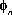arrive from an angle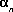relative to the direction of the motion of the antenna.The Doppler shift of this wave is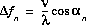,

where v is the speed of the antenna.

## Phasor representation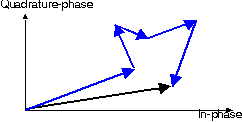Figure: Phasor diagram of a set of scattered waves (in blue), resulting a Rayleigh-fading envelope (in black). Animation.

In case of an unmodulated carrier, the transmitted signal has the form.

The received unmodulated signal r(t) can be expressed as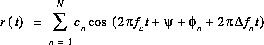An inphase-quadrature representation of the form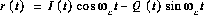can be found with in-phase component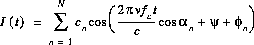.

### (Quasi-) Stationary Channel Snapshot

Let's consider a stationary user, thus v=0. An inphase-quadrature representation reduces toandThus both the inphase and quadrature component, I(t) and Q(t) can be interpreted as the sum of many (independent) small contributions. Each contribution is due to a particular reflection, with its own amplitude cn and phase. For sufficiently many reflections (large N), the Central Limit Theorem now says that the inphase and quadrature components tend to a Gaussian distribution of their amplitude. I(t) and Q(t) appear to be independent and identically distributed (iid).

Note also that if the antenna speed is set to zero, channel fluctuations no longer occur. Fading is due to motion of the antenna. An exception occurs if reflecting objects move. In a vehicular cellular phone system, the user is likely to move out of a fade, but in a Wireless LAN, a terminal may by accident be placed permanently in a fade where no reliable coverage is available.

### Elaboration of this model

 Parameter Probability Distribution I(t1) and Q(t1) components i.i.d. Gaussian, Zero mean I(t1) and Q(t2) components at time offset Correlated jointly Gaussian I(t2) given I(t1) Gaussian Amplitude r Rayleigh r(t2) given r(t1) Rician Derivative of Amplitude Gaussian Independent of amplitude Phase Uniform Derivative of Phase Gaussian Dependent on amplitude Power exponential

This propagation model allows us, for instance,

If the set of reflected waves are dominated by one strong component, Rician fading is a more appropriate model.